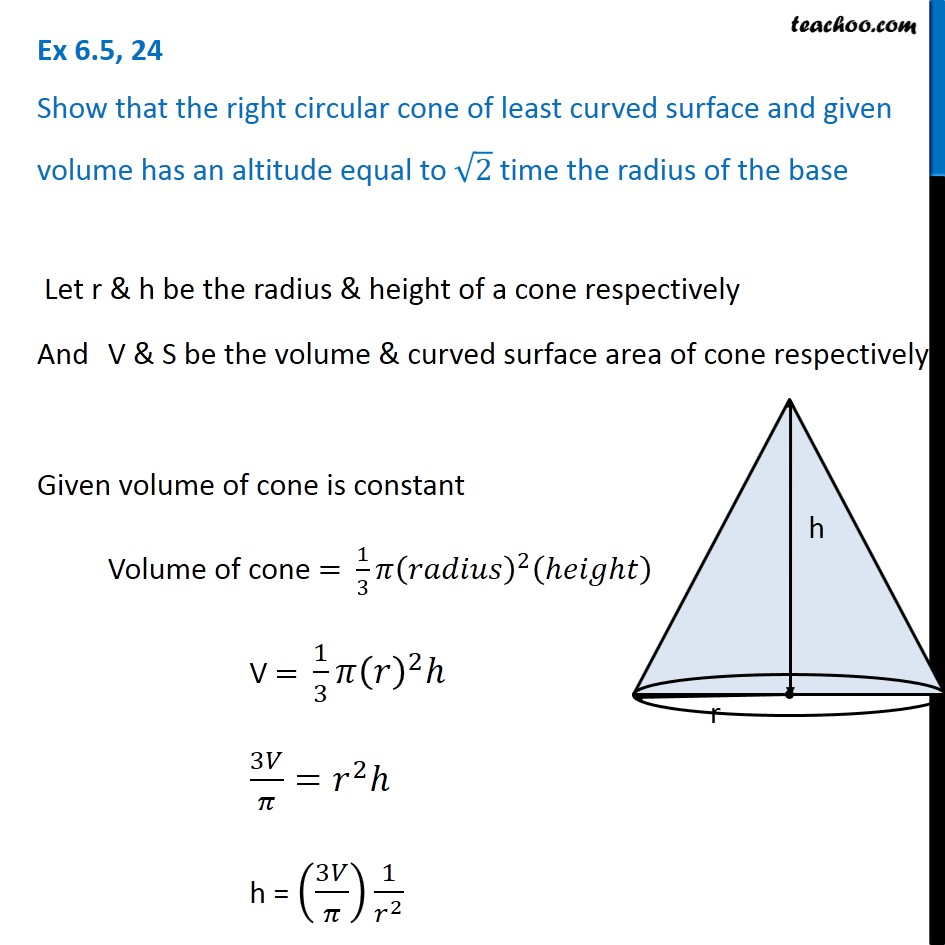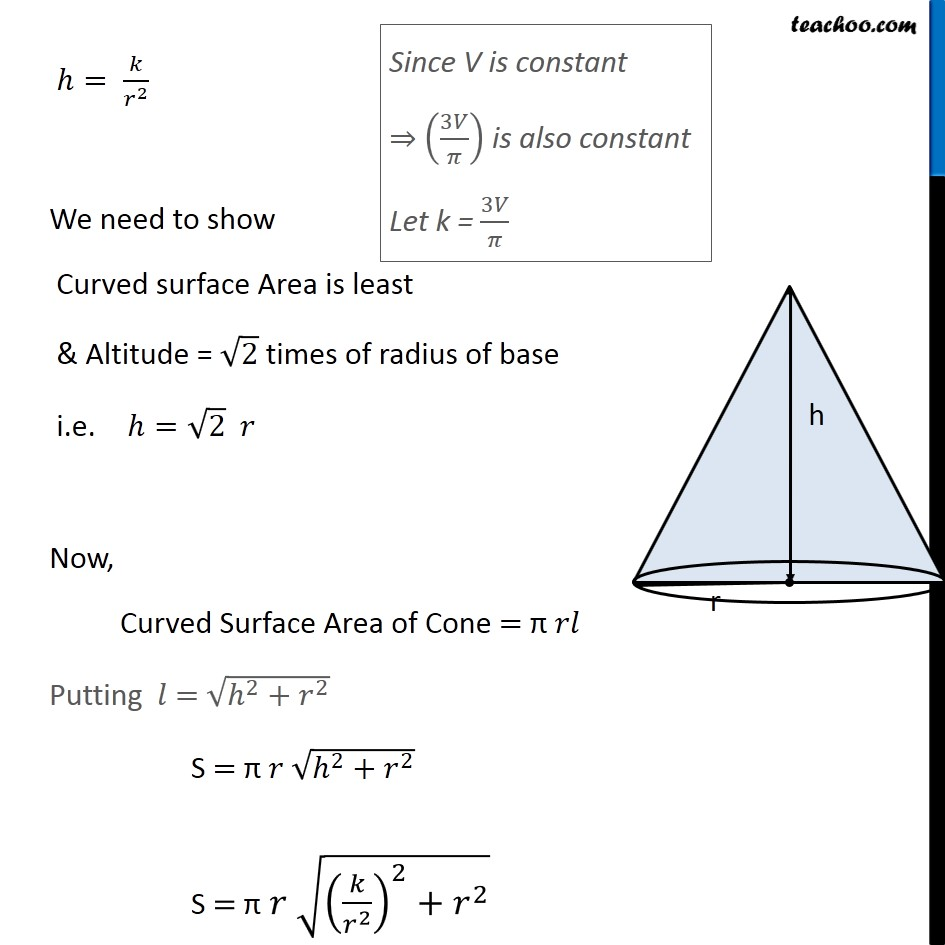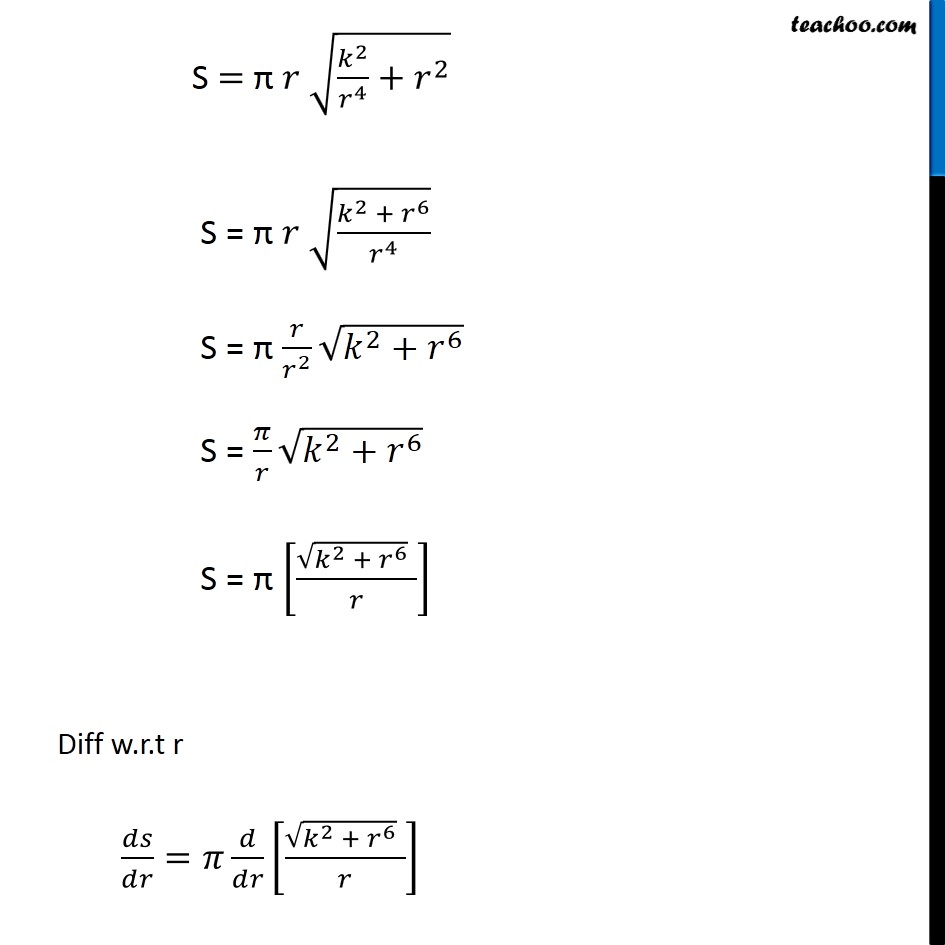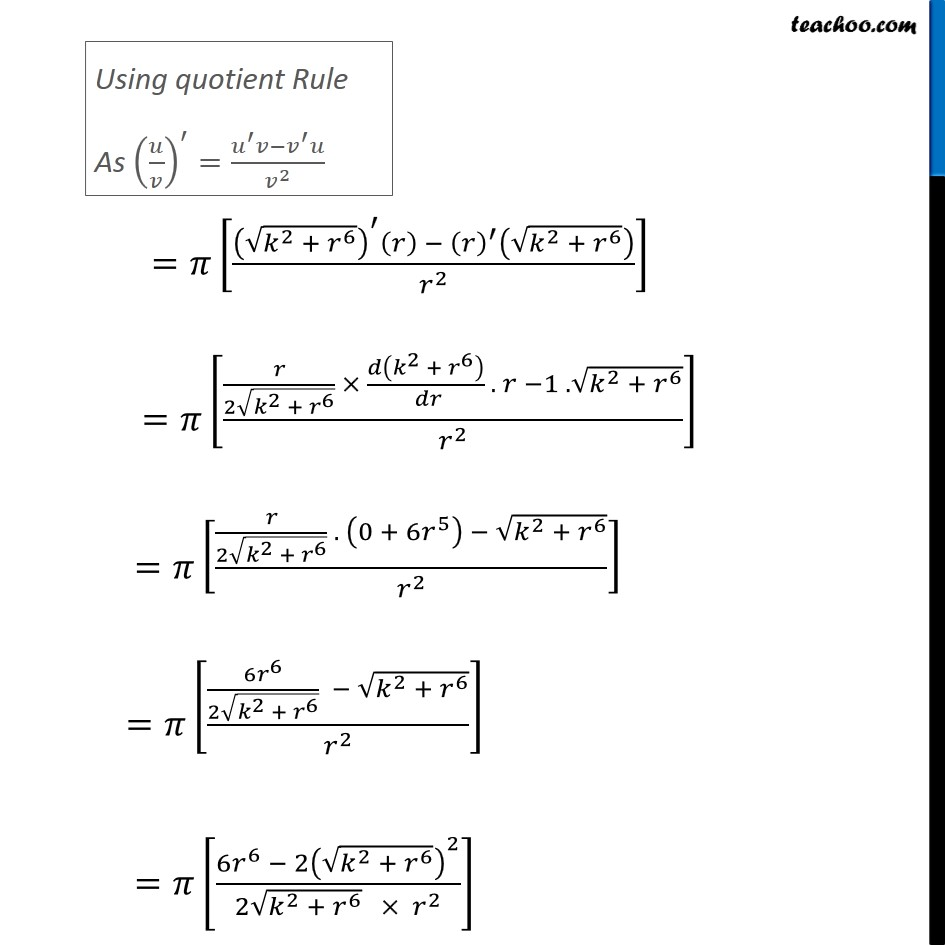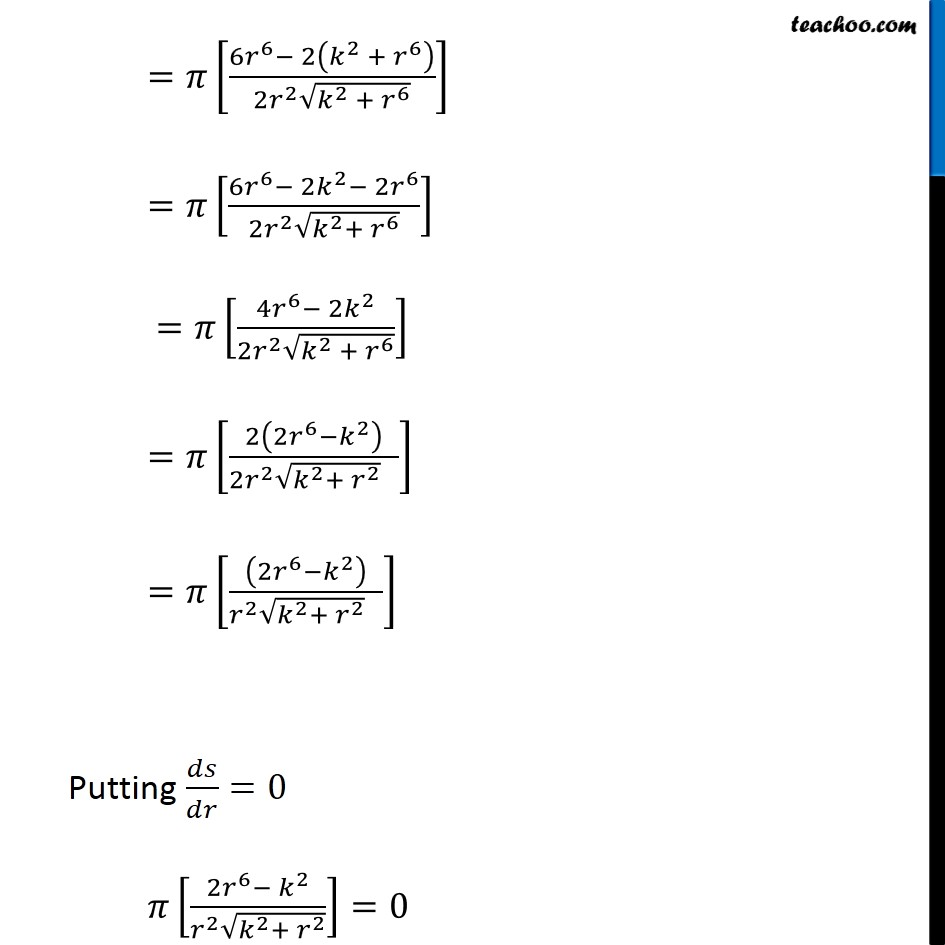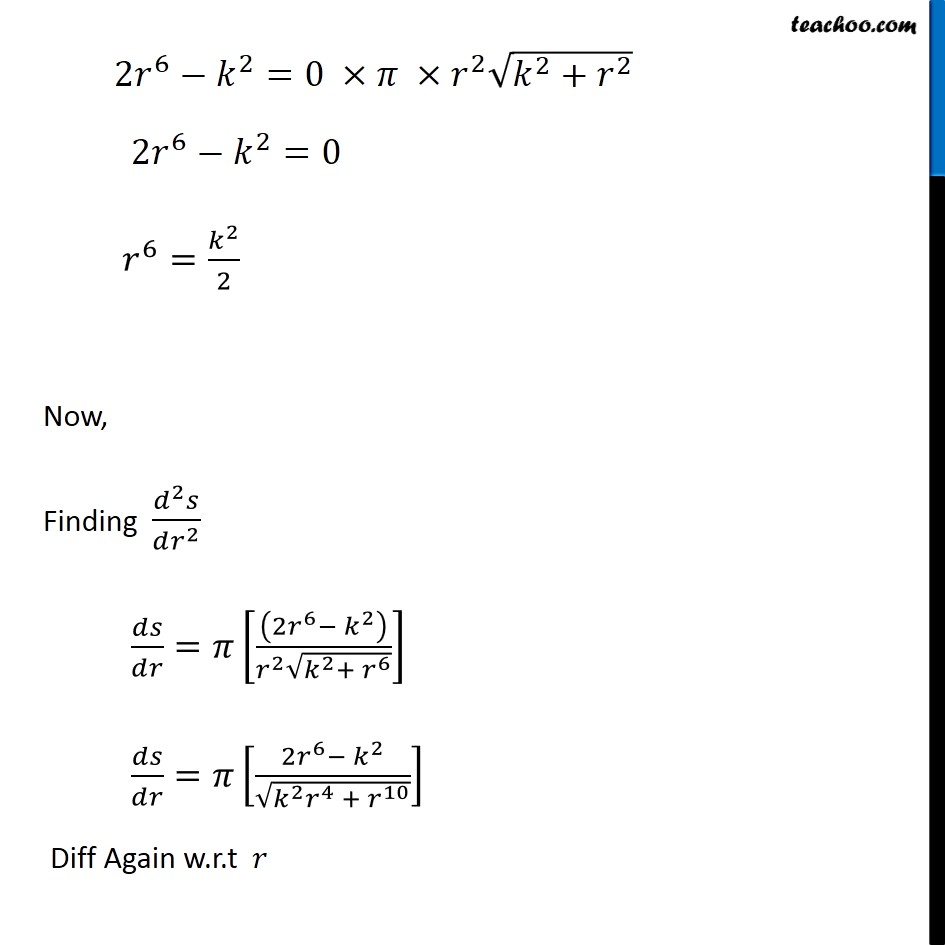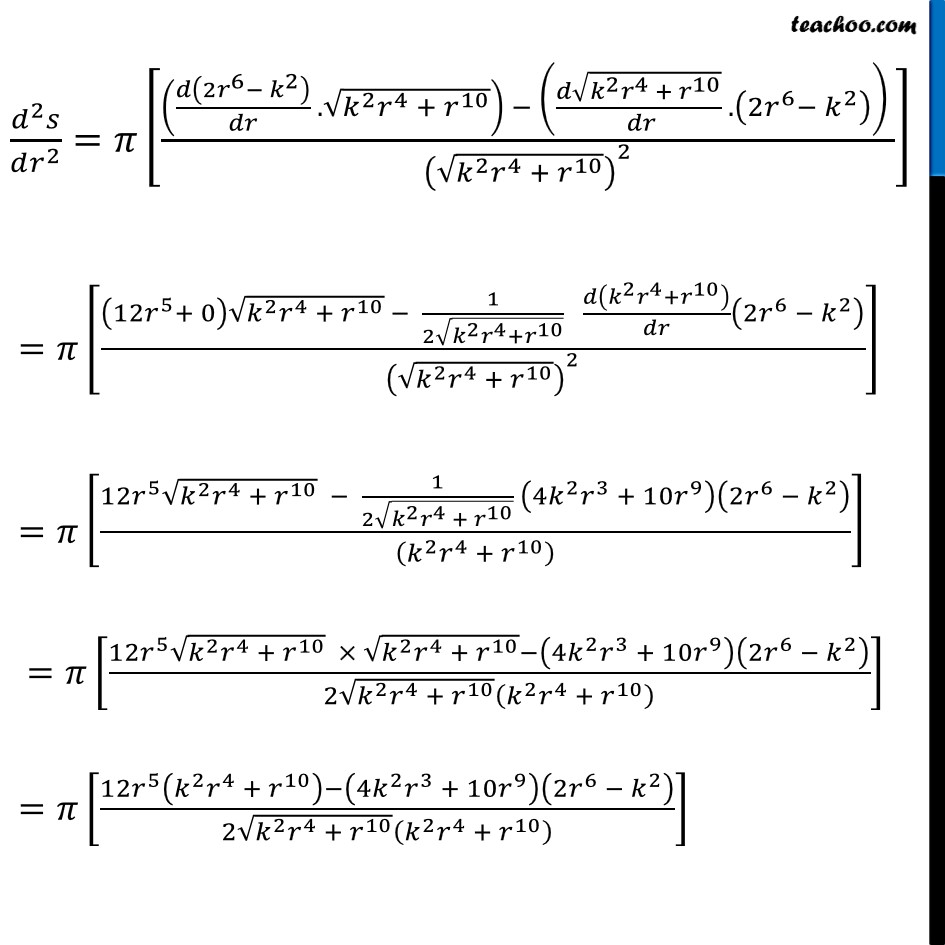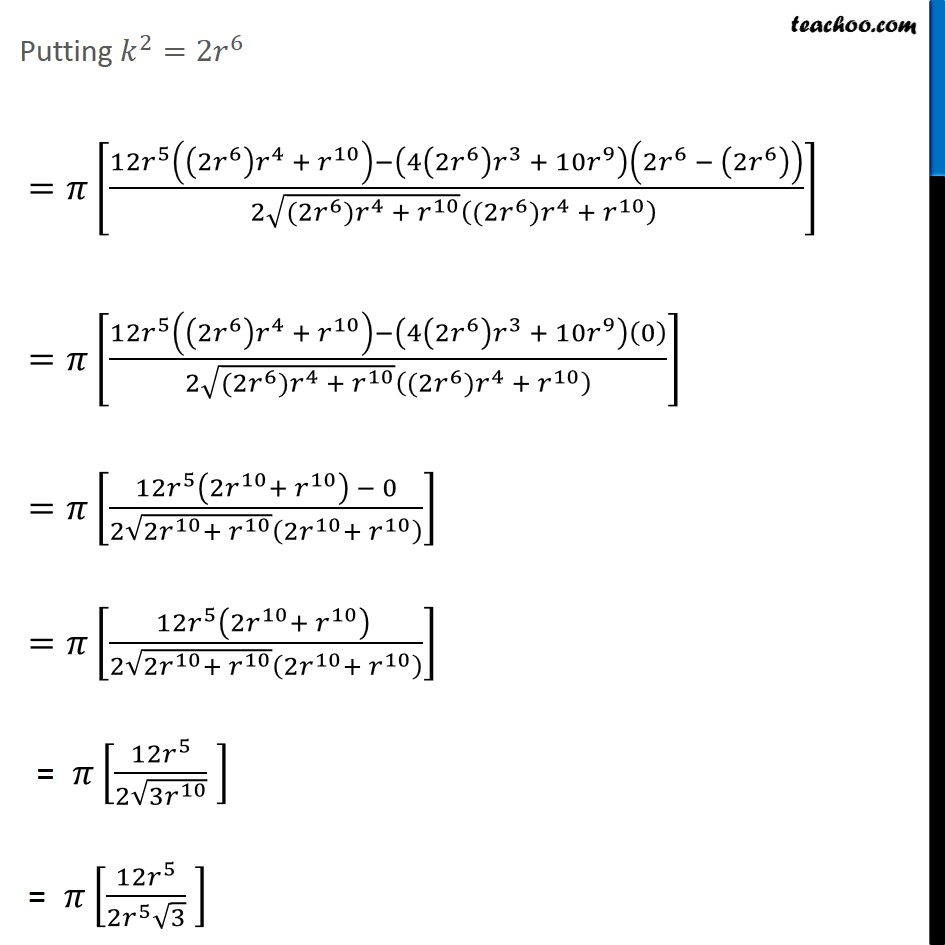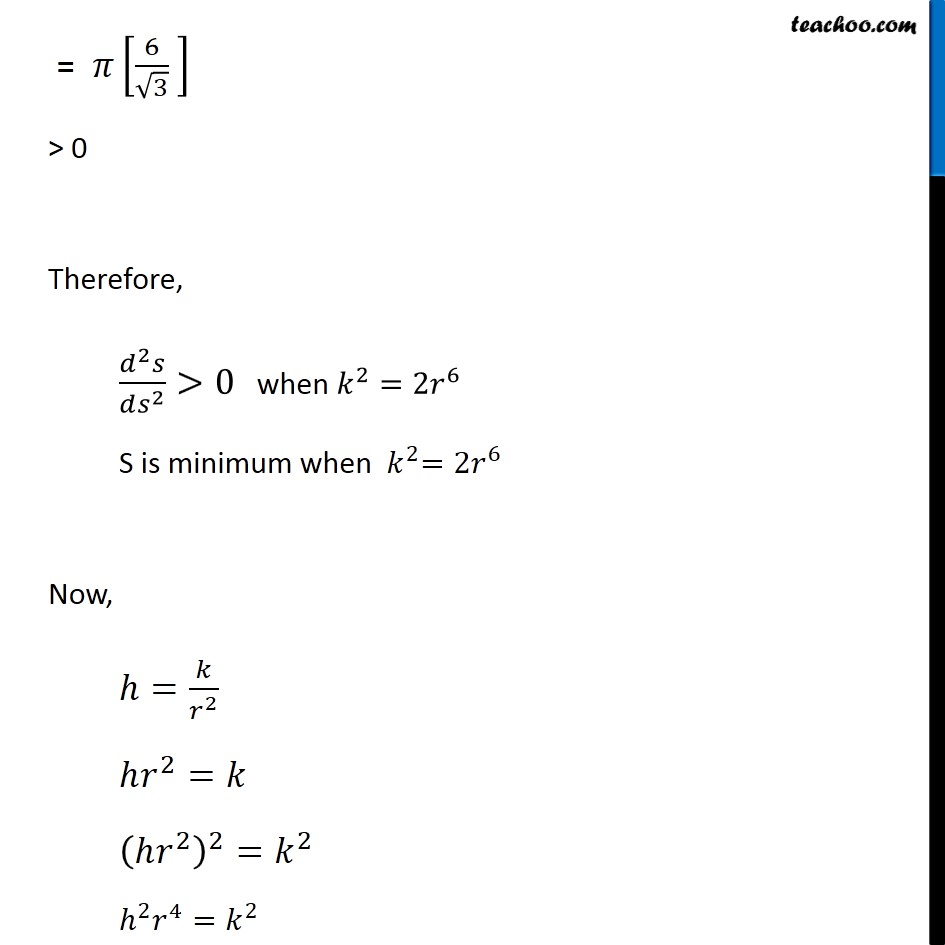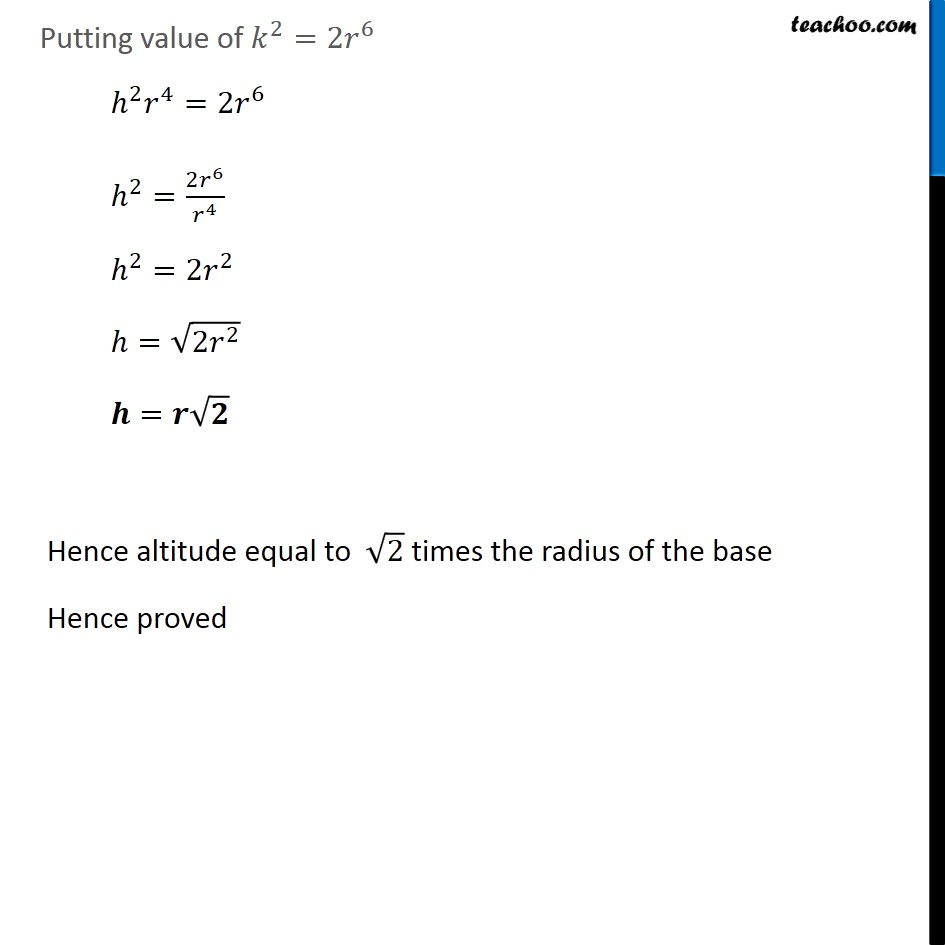1. Chapter 6 Class 12 Application of Derivatives
2. Serial order wise
3. Ex 6.5

Transcript

Ex 6.5, 24 Show that the right circular cone of least curved surface and given volume has an altitude equal to √2 time the radius of the base Let r & h be the radius & height of a cone respectively And V & S be the volume & curved surface area of cone respectively Given volume of cone is constant Volume of cone = 1/3 𝜋(𝑟𝑎𝑑𝑖𝑢𝑠)^2 (ℎ𝑒𝑖𝑔ℎ𝑡) V = 1/3 𝜋(𝑟)^2 ℎ 3𝑉/𝜋=𝑟^2 ℎ h = (3𝑉/𝜋) 1/𝑟^2 ℎ= 𝑘/𝑟^2 We need to show Curved surface Area is least & Altitude = √2 times of radius of base i.e. ℎ=√2 𝑟 Now, Curved Surface Area of Cone = π 𝑟𝑙 Putting 𝑙 = √(ℎ^2+𝑟^2 ) S = π 𝑟 √(ℎ^2+𝑟^2 ) S = π 𝑟 √((𝑘/𝑟^2 )^2+𝑟^2 ) S = π 𝑟 √(𝑘^2/𝑟^4 +𝑟^2 ) S = π 𝑟 √((𝑘^2 + 𝑟^6)/𝑟^4 ) S = π 𝑟/𝑟^2 √(𝑘^2+𝑟^6 ) S = 𝜋/𝑟 √(𝑘^2+𝑟^6 ) S = π [(√(𝑘^2 + 𝑟^6 ) " " )/𝑟] Diff w.r.t r 𝑑𝑠/𝑑𝑟=𝜋 𝑑/𝑑𝑟 [(√(𝑘^2 + 𝑟^6 ) " " )/𝑟] =𝜋[((√(𝑘^2 + 𝑟^6 ))^′ (𝑟) − (𝑟)^′ (√(𝑘^2 + 𝑟^6 )))/𝑟^2 ] =𝜋[(𝑟/(2√(𝑘^2 + 𝑟^6 )) × 𝑑(𝑘^2 + 𝑟^6 )/( 𝑑𝑟) . 𝑟 −1 .√(𝑘^2 + 𝑟^6 ))/𝑟^2 ] =𝜋[(𝑟/(2√(𝑘^2 + 𝑟^6 )) . (0 + 6𝑟^5 ) − √(𝑘^2 + 𝑟^6 ))/𝑟^2 ] =𝜋[((6𝑟^6)/(2√(𝑘^2 + 𝑟^6 )) − √(𝑘^2 + 𝑟^6 ))/𝑟^2 ] =𝜋[(6𝑟^6 − 2(√(𝑘^2 + 𝑟^6 ))^2)/(2√(𝑘^2 + 𝑟^6 ) × 𝑟^2 )] =𝜋[(6𝑟^6− 2(𝑘^2 + 𝑟^6 ))/(2𝑟^2 √(𝑘^2 + 𝑟^6 ))] =𝜋[(6𝑟^6− 2𝑘^2− 2𝑟^6)/(2𝑟^2 √(𝑘^2+〖 𝑟〗^6 ))] =𝜋[(4𝑟^6− 2𝑘^2)/(2𝑟^2 √(𝑘^2 + 𝑟^6 ))] =𝜋[2(2𝑟^6−𝑘^2 )/(2𝑟^2 √(𝑘^2+ 𝑟^2 ) )] =𝜋[((2𝑟^6−𝑘^2 ))/(𝑟^2 √(𝑘^2+ 𝑟^2 ) )] Putting 𝑑𝑠/𝑑𝑟=0 𝜋[(2𝑟^6− 𝑘^2)/(𝑟^2 √(𝑘^2+ 𝑟^2 ))]=0 2𝑟^6−𝑘^2=0 ×𝜋 ×𝑟^2 √(𝑘^2+𝑟^2 ) 2𝑟^6−𝑘^2=0 𝑟^6=𝑘^2/2 Now, Finding (𝑑^2 𝑠)/(𝑑𝑟^2 ) 𝑑𝑠/𝑑𝑟=𝜋[((2𝑟^6− 𝑘^2 ))/(𝑟^2 √(𝑘^2+ 𝑟^6 ))] 𝑑𝑠/𝑑𝑟=𝜋[(2𝑟^6− 𝑘^2)/√(𝑘^2 𝑟^4 + 𝑟^10 )] Diff Again w.r.t 𝑟 (𝑑^2 𝑠)/(𝑑𝑟^2 )=𝜋[((𝑑(2𝑟^6− 𝑘^2 )/𝑑𝑟 .√(𝑘^2 𝑟^4 + 𝑟^10 )) − ((𝑑√(𝑘^2 𝑟^4 + 𝑟^10 ))/( 𝑑𝑟) . (2𝑟^6− 𝑘^2 )) )/(√(𝑘^2 𝑟^4 + 𝑟^10 ))^2 ] =𝜋[((12𝑟^5+ 0) √(𝑘^2 𝑟^4 + 𝑟^10 ) − 1/(2√(𝑘^2 𝑟^4+𝑟^10 )) 𝑑(𝑘^2 𝑟^4+𝑟^10 )/𝑑𝑟 (2𝑟^6 − 𝑘^2 ))/(√(𝑘^2 𝑟^4 + 𝑟^10 ))^2 ] =𝜋[(12𝑟^5 √(𝑘^2 𝑟^4 + 𝑟^10 ) − 1/(2√(𝑘^2 𝑟^4 + 𝑟^10 )) (4𝑘^2 𝑟^3 + 10𝑟^9 )(2𝑟^6 − 𝑘^2 ))/((𝑘^2 𝑟^4 + 𝑟^10 ) )] =𝜋[(12𝑟^5 √(𝑘^2 𝑟^4 + 𝑟^10 ) × √(𝑘^2 𝑟^4 + 𝑟^10 )−(4𝑘^2 𝑟^3 + 10𝑟^9 )(2𝑟^6 − 𝑘^2 ))/(2√(𝑘^2 𝑟^4 + 𝑟^10 ) (𝑘^2 𝑟^4 + 𝑟^10 ) )] =𝜋[(12𝑟^5 (𝑘^2 𝑟^4 + 𝑟^10 )−(4𝑘^2 𝑟^3 + 10𝑟^9 )(2𝑟^6 − 𝑘^2 ))/(2√(𝑘^2 𝑟^4 + 𝑟^10 ) (𝑘^2 𝑟^4 + 𝑟^10 ) )] Putting 𝑘^2=2𝑟^6 =𝜋[(12𝑟^5 ((2𝑟^6 ) 𝑟^4 + 𝑟^10 )−(4(2𝑟^6 ) 𝑟^3 + 10𝑟^9 )(2𝑟^6 − (2𝑟^6 )))/(2√((2𝑟^6)𝑟^4 + 𝑟^10 ) ((2𝑟^6)𝑟^4 + 𝑟^10 ) )] =𝜋[(12𝑟^5 ((2𝑟^6 ) 𝑟^4 + 𝑟^10 )−(4(2𝑟^6 ) 𝑟^3 + 10𝑟^9 )(0))/(2√((2𝑟^6)𝑟^4 + 𝑟^10 ) ((2𝑟^6)𝑟^4 + 𝑟^10 ) )] =𝜋[(12𝑟^5 (2𝑟^10+ 𝑟^10 ) − 0)/(2√(2𝑟^10+ 𝑟^10 ) (2𝑟^10+ 𝑟^10 ) )] =𝜋[(12𝑟^5 (2𝑟^10+ 𝑟^10 ) )/(2√(2𝑟^10+ 𝑟^10 ) (2𝑟^10+ 𝑟^10 ) )] = 𝜋[(12𝑟^5)/(2√(3𝑟^10 )) ] = 𝜋[(12𝑟^5)/(2𝑟^5 √3) ] = 𝜋[6/√3 ] > 0 Therefore, (𝑑^2 𝑠)/(𝑑𝑠^2 )>0 when 𝑘^2=2𝑟^6 S is minimum when 〖 𝑘〗^2=2𝑟^6 Now, ℎ=𝑘/𝑟^2 ℎ𝑟^2=𝑘 (ℎ𝑟^2 )^2=𝑘^2 ℎ^2 𝑟^4=𝑘^2 Putting value of 𝑘^2=2𝑟^6 ℎ^2 𝑟^4=2𝑟^6 ℎ^2=(2𝑟^6)/𝑟^4 ℎ^2=2𝑟^2 ℎ=√(2𝑟^2 ) 𝒉=𝒓√𝟐 Hence altitude equal to √2 times the radius of the base Hence proved

Ex 6.5